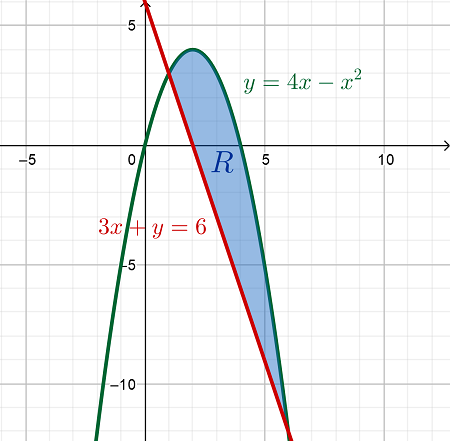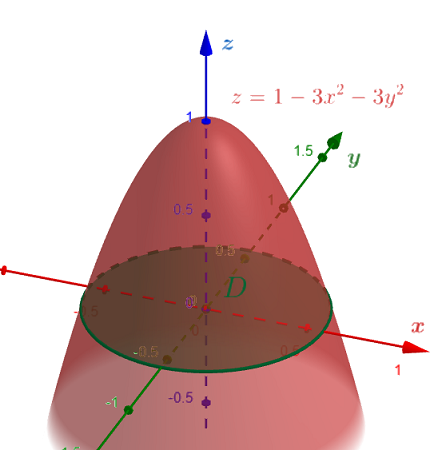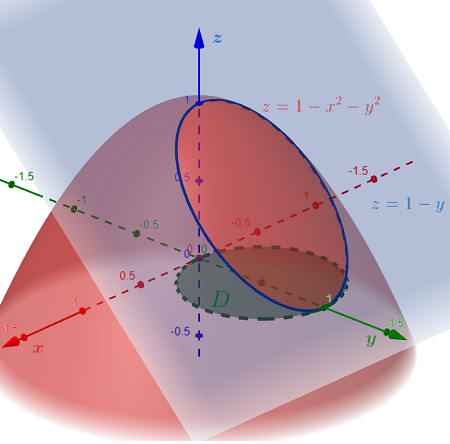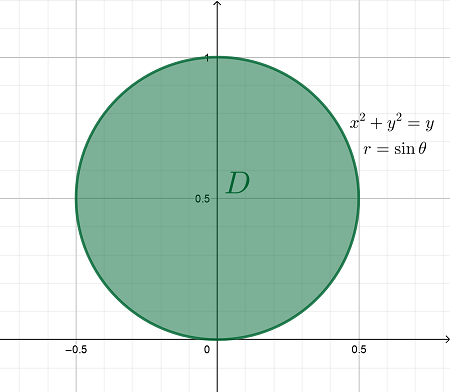# 1. Use a double integral to find the area of the region that lies below the parabola y = 4x -...

## Question:

1. Use a double integral to find the area of the region that lies below the parabola {eq}y = 4x - x^2 {/eq} and above the line 3x + y = 6.

2. Find the volume under the paraboloid {eq}z = 1 - 3x^2 - 3y^2 {/eq} and above the xy-plane.

3. Find the volume of the region bounded above by the paraboloid {eq}z = 1 - x^2 - y^2 {/eq} and below by the plane z = 1 - y.

## Volumes of Solids:

The volume of any solid {eq}E {/eq} in {eq}\mathbb{R}^3 {/eq} can be calculated using the triple integral:

$$Volume = \iiint \limits_E dV$$

However, suppose the solid {eq}E {/eq} is described as being the solid between two smooth surfaces {eq}z = F(x,y) \ge G(x,y) {/eq} over the planar region {eq}D {/eq} on the xy-plane.

Then, the volume of the solid can be expressed using only double integrals such that:

$$Volume = \iint \limits_D \left[ F(x,y) - G(x,y) \right] \,dA$$

1. Let {eq}R {/eq} be the given region which lies below the parabola {eq}y = 4x - x^2 {/eq} and above the line {eq}3x + y = 6 {/eq}.Determine the x-coordinates of the intersection points of the parabola and the line:

{eq}\begin{align*} &y = 4x - x^2 = 6 - 3x & \text{[Solve for } x ] \\ &\Rightarrow x^2 - 7x + 6 = 0 \\ &\Rightarrow (x - 1) (x - 6) = 0 \\ &\Rightarrow x - 1 = 0 \text{ or } x - 6 = 0 \\ &\Rightarrow x = 1 \text{ or } x = 6 \end{align*} {/eq}

Based on the graph, the given region {eq}R {/eq} can be described using the inequalities {eq}1 \le x \le 6 \text{ and } 6 - 3x \le y \le 4x - x^2 {/eq}. Then, the area of the region {eq}R {/eq} is given by the double integrals:

{eq}\begin{align*} Area &= \iint \limits_R dA & \text{[Evaluate as iterated integrals]} \\ &= \int_1^6 \int_{6 - 3x}^{4x - x^2} dy \,dx & \text{[Integrate with respect to } y \text{]} \\ &= \int_1^6 \left. y \right|_{6 - 3x}^{4x - x^2} \,dx & \text{[Fundamental Theorem of Calculus]} \\ &= \int_1^6 \left[ ( 4x - x^2 ) - ( 6 - 3x ) \right] \,dx \\ &= \int_1^6 ( -x^2 + 7x - 6 ) \,dx & \text{[Integrate with respect to } x \text{]} \\ &= \left. \left( - \frac{x^3}{3} + \frac{7x^2}{2} - 6x \right) \right|_1^6 & \text{[Fundamental Theorem of Calculus]} \\ &= \left[ - \frac{6^3}{3} + \frac{7 (6)^2}{2} - 6(6) \right] - \left[ - \frac{1^3}{3} + \frac{7 (1)^2}{2} - 6(1) \right] & \text{[Simplify]} \\ &= ( -72 + 126 - 36 ) - \left( - \frac{1}{3} + \frac{7}{2} - 6 \right) \\ &= 18 + \frac{17}{6} \\ Area &= \boxed{ \frac{125}{6} } & \boxed{ \text{Area of the Bounded Region } R } \end{align*} {/eq}

Note that the integral above is similar to the double integrals for the volume of a solid, only that the integrand above is equal to {eq}1 {/eq}.

This is because if the solid is a prism that is bounded above by the plane {eq}z = 1 {/eq} and below by the region {eq}R {/eq}, the solid will have {eq}R {/eq} as its base and height equal to {eq}h =1 {/eq}. Then, the volume of such solid is equal to:

{eq}\begin{align*} &Volume = \iint \limits_R 1 \,dA = Area_{base} \times h \\ &Volume = \iint \limits_R dA = Area_R \end{align*} {/eq}

2. Volume under the paraboloid {eq}z = 1 - 3x^2 - 3y^2 {/eq} and above the xy-plane. Let {eq}D {/eq} be the planar region on the xy-plane that is under the given solid.Determine the equation of the intersection of the paraboloid and the xy-plane, which encloses the region {eq}D {/eq}.

{eq}\begin{align*} &z = 1 - 3x^2 - 3y^2 = 0 \\ &\Rightarrow 1 - 3x^2 - 3y^2 = 0 \\ &\Rightarrow 3x^2 + 3y^2 = 1 \\ &\Rightarrow x^2 + y^2 = \frac{1}{3} & \text{[Equation of a circle. Convert into its polar form using } x^2 + y^2 = r^2 ] \\ &\Rightarrow r^2 = \frac{1}{3} & \text{[Take the positive root of } r ] \\ &\Rightarrow r = \frac{1}{\sqrt{3}} \end{align*} {/eq}

Since the region {eq}D {/eq} is circular, it is better to define it in polar coordinates such that {eq}D = \left\{\, (r,\theta) \,|\, 0 \le r \le \frac{1}{\sqrt{3}} \text{ and } 0 \le \theta \le 2\pi \,\right\} {/eq}.Therefore, the volume of the solid under the paraboid and over the region {eq}D {/eq} is given by the integral:

{eq}\begin{align*} Volume &= \iint \limits_D ( 1 - 3x^2 - 3y^2 ) \,dA & \text{[Evaluate as iterated integrals in polar coordinates such that } dA = r \,dr \,d\theta ] \\ &= \int_0^{2\pi} \int_0^\frac{1}{\sqrt{3}} (1 - 3r^2 ) \,r \,dr \,d\theta & \text{[Since } x^2 + y^2 = r^2 ] \\ &= \int_0^{2\pi} \int_0^\frac{1}{\sqrt{3}} (r - 3r^3) \,dr \,d\theta & \text{[Integrate with respect to } r \text{]} \\ &= \int_0^{2\pi} \left. \left( \frac{r^2}{2} - \frac{3r^4}{4} \right) \right|_0^\frac{1}{\sqrt{3}} \,d\theta & \text{[Fundamental Theorem of Calculus]} \\ &= \int_0^{2\pi} \left( \left[ \frac{1}{2} \left( \frac{1}{\sqrt{3}} \right)^2 - \frac{3}{4} \left( \frac{1}{\sqrt{3}} \right)^4 \right] - 0 \right) \,d\theta \\ &= \int_0^{2\pi} \left( \frac{1}{6} - \frac{1}{12} \right) \,d\theta \\ &= \int_0^{2\pi} \frac{1}{12} \,d\theta & \text{[Integrate with respect to } \theta \text{]} \\ &= \left. \frac{1}{12} \theta \right|_0^{2\pi} & \text{[Fundamental Theorem of Calculus]} \\ &= \frac{1}{12} (2\pi - 0) \\ Volume &= \boxed{ \frac{\pi}{6} } & \boxed{ \text{Volume of the Solid under the Paraboloid} } \end{align*} {/eq}

3. Volume under the paraboloid {eq}z = 1 - x^2 - y^2 {/eq} and above the xy-plane and below by the plane {eq}z = 1 - y {/eq}. Let {eq}D {/eq} be the planar region on the xy-plane that is under the given solid.Solve for the equation of the intersection of the paraboloid and the plane:

{eq}\begin{align*} &z = 1 - x^2 - y^2 = 1 - y \\ &\Rightarrow 1 - x^2 - y^2 = 1 - y \\ &\Rightarrow x^2 + y^2 = y & \text{[Equation 1]} \\ \\ &\Rightarrow x^2 + \left( y^2 - y + \frac{1}{4} \right) = \frac{1}{4} \\ &\Rightarrow x^2 + \left( y - \frac{1}{2} \right)^2 = \left( \frac{1}{2} \right)^2 & \left[ \text{Equation of a circle centered at } \left( 0 , \frac{1}{2} \right) \text{ with radius } r = \frac{1}{2} \right] \\ \\ &\Rightarrow x^2 + y^2 = y & \text{[Convert into its polar form using the identity } x^2 + y^2 = (r \cos \theta)^2 + (r \sin \theta)^2 = r^2 ] \\ &\Rightarrow r^2 = r \sin \theta \\ &\Rightarrow r = \sin \theta & \text{[Polar equation of the circle]} \end{align*} {/eq}

Similar to Item 2, since the region {eq}D {/eq} is a circular region, it is better to define the region in polar coordinates such that {eq}D = \left\{\, (r,\theta) \,|\, 0 \le r \le \sin \theta \text{ and } 0 \le \theta \le 2\pi \,\right\} {/eq}.Therefore, the volume of the bounded solid over the region {eq}D {/eq} is given by the integral:

{eq}\begin{align*} Volume &= \iint \limits_D \left[ ( 1 - x^2 - y^2 ) - ( 1 - y ) \right] \,dA & \text{[Convert the integrand into its polar form]} \\ &= \iint \limits_D ( y - x^2 - y^2 ) \,dA \\ &= \iint \limits_D ( r \sin \theta - r^2 ) \,dA & \text{[Evaluate as iterated integrals in polar coordinates]} \\ &= \int_0^{2\pi} \int_0^{\sin\theta} (r \sin \theta - r^2) \,r \,dr \,d\theta \\ &= \int_0^{2\pi} \int_0^{\sin\theta} (r^2 \sin \theta - r^3) \,dr \,d\theta & \text{[Integrate with respect to } r \text{]} \\ &= \int_0^{2\pi} \left. \left( \frac{r^3}{3} \sin \theta - \frac{r^4}{4} \right) \right|_0^{\sin\theta} \,d\theta & \text{[Fundamental Theorem of Calculus]} \\ &= \int_0^{2\pi} \left[ \left( \frac{\sin^3 \theta}{3} \sin \theta - \frac{\sin^4 \theta}{4} \right) - 0 \right] \,d\theta \\ &= \int_0^{2\pi} \frac{1}{12} \sin^4 \theta \,d\theta \\ &= \int_0^{2\pi} \frac{1}{12} \left( \sin^2 \theta \right)^2 \,d\theta & \left[ \text{Use the double-angle identity } \sin^2 \theta = \frac{1}{2} (1 - \cos 2\theta) \right] \\ &= \int_0^{2\pi} \frac{1}{48} \left[ \frac{1}{2} (1 - \cos 2\theta) \right]^2 \,d\theta \\ &= \int_0^{2\pi} \frac{1}{48} (1 - \cos 2\theta)^2 \,d\theta \\ &= \int_0^{2\pi} \frac{1}{48} (\cos^2 2\theta - 2 \cos 2\theta + 1) \,d\theta & \left[ \text{Use the double-angle identity } \cos^2 \theta = \frac{1}{2} (1 + \cos 2\theta) \right] \\ &= \int_0^{2\pi} \frac{1}{48} \left( \frac{1}{2} + \frac{1}{2} \cos 4\theta - 2 \cos 2\theta + 1 \right) \,d\theta \\ &= \int_0^{2\pi} \frac{1}{96} ( \cos 4\theta - 4 \cos 2\theta + 3 ) \,d\theta & \left[ \text{For all constant } k \,,\, \int k \cdot f(x) \,dx = k \int f(x)\,dx \right] \\ &= \frac{1}{96} \int_0^{2\pi} ( \cos 4\theta - 4 \cos 2\theta + 3 ) \,d\theta & \text{[Integrate with respect to } \theta \text{]} \\ &= \frac{1}{96} \left. \left( \frac{1}{4} \sin 4\theta - 2 \sin 2\theta + 3\theta \right) \right|_0^{2\pi} & \text{[Fundamental Theorem of Calculus]} \\ &= \frac{1}{96} \left( \left[ \frac{1}{4} \sin 4 (2\pi) - 2 \sin 2(2\pi) + 3(2\pi) \right] - \left[ \frac{1}{4} \sin 0 - 2 \sin 0 + 0 \right] \right) & \text{[Simplify]} \\ &= \frac{1}{96} \left[ \left( 0 - 0 + 6\pi \right) - 0 \right] \\ Volume &= \boxed{ \frac{\pi}{16} } & \boxed{ \text{Volume of the Bounded Solid} } \end{align*} {/eq}